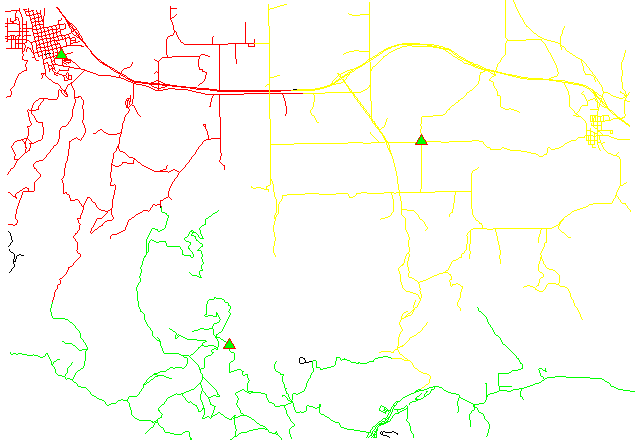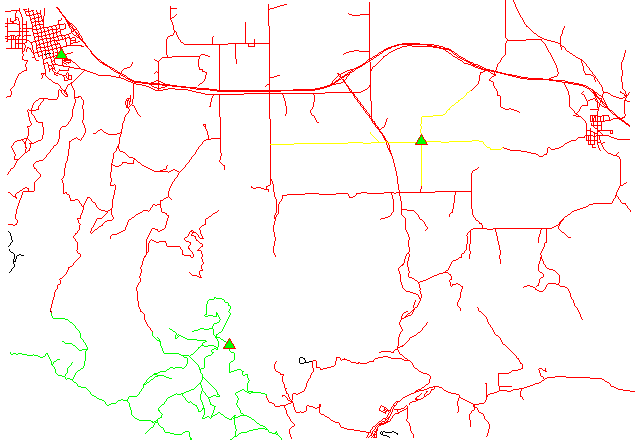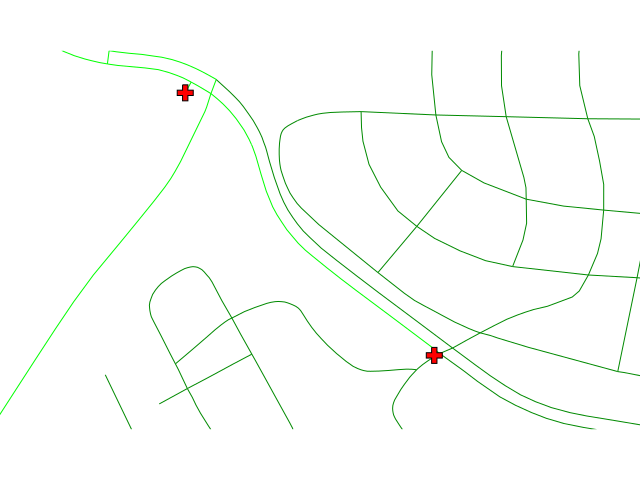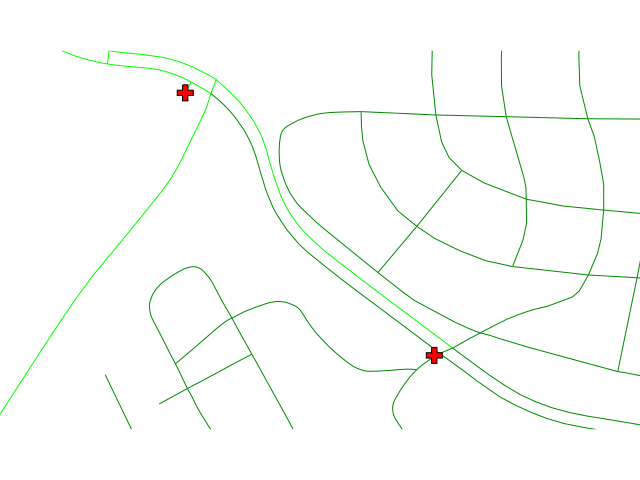Note: This document is for an older version of GRASS GIS that will be discontinued soon. You should upgrade, and read the current manual page.

## NAME

v.net.alloc - Allocates subnets for nearest centers.
Center node must be opened (costs >= 0). Costs of center node are used in calculation.

## KEYWORDS

vector, network, cost allocation

## SYNOPSIS

v.net.alloc
v.net.alloc --help
v.net.alloc [-tgu] input=name output=name [method=string] center_cats=range arc_layer=string arc_type=string[,string,...] node_layer=string [arc_column=name] [arc_backward_column=name] [node_column=name] [turn_layer=string] [turn_cat_layer=string] [--overwrite] [--help] [--verbose] [--quiet] [--ui]

### Flags:

-t
Use turntable
-g
Use geodesic calculation for longitude-latitude locations
-u
Create unique categories and attribute table
Default: same category like nearest center
--overwrite
Allow output files to overwrite existing files
--help
Print usage summary
--verbose
Verbose module output
--quiet
Quiet module output
--ui
Force launching GUI dialog

### Parameters:

input=name [required]
Name of input vector map
Or data source for direct OGR access
output=name [required]
Name for output vector map
method=string
Use costs from centers or costs to centers
Options: from, to
Default: from
center_cats=range [required]
Category values
Categories of centers (points on nodes) to which net will be allocated, layer for this categories is given by nlayer option
arc_layer=string [required]
Arc layer
Vector features can have category values in different layers. This number determines which layer to use. When used with direct OGR access this is the layer name.
Default: 1
arc_type=string[,string,...] [required]
Arc type
Input feature type
Options: line, boundary
Default: line,boundary
node_layer=string [required]
Node layer
Vector features can have category values in different layers. This number determines which layer to use. When used with direct OGR access this is the layer name.
Default: 2
arc_column=name
Arc forward/both direction(s) cost column (number)
arc_backward_column=name
Arc backward direction cost column (number)
node_column=name
Node cost column (number)
turn_layer=string
Layer with turntable
Relevant only with -t flag
Default: 3
turn_cat_layer=string
Layer with unique categories used in turntable
Relevant only with -t flag
Default: 4

## DESCRIPTION

v.net.alloc allocates subnets for nearest centers. Center nodes must be opened (costs >= 0). Costs of center nodes are used in the calculation.

Costs may be either line lengths, or attributes saved in a database table. These attribute values are taken as costs of whole segments, not as costs to traverse a length unit (e.g. meter) of the segment. For example, if the speed limit is 100 km / h, the cost to traverse a 10 km long road segment must be calculated as
length / speed = 10 km / (100 km/h) = 0.1 h.
Supported are cost assignments for both arcs and nodes, and also different costs for both directions of a vector line. For areas, costs will be calculated along boundary lines.

The input vector needs to be prepared with v.net operation=connect in order to connect points representing center nodes to the network.

The nearest center can be determined using either costs from the nearest center or costs to the nearest center with option method. See example below.

By default, the category value of the nearest center is used as category value for output lines. With the -u flag, output lines become unique categories and an attribute table is created with the fields cat, ocat, center. The ocat field holds the original line category in arc_layer and the center field holds the center category in node_layer. Additionally, original line categories are copied from the input arc_layer to layer 2 in the output, together with any attribute table.

Application of flag -t enables a turntable support. This flag requires additional parameters turn_layer and turn_cat_layer that are otherwise ignored. The turntable allows to model e.g. traffic code, where some turns may be prohibited. This means that the input layer is expanded by turntable with costs of every possible turn on any possible node (intersection) in both directions. Turntable can be created by the v.net module. For more information about turns in the vector network analyses see wiki page.

## NOTES

Nodes and arcs can be closed using cost = -1.

Center nodes can also be assigned to vector nodes using wxGUI vector digitizer.

## EXAMPLES

1. Subnetwork allocation using distance:2. Subnetwork allocation using traveling time:Example 1: Calculating subnets for 3 center nodes using distances

```# Spearfish

# center nodes:
echo "591235.5|4926306.62|1
596591.8|4917042.5|2
602722.9|4923544.2|3" | v.in.ascii in=- out=centernodes

# connect points to network

# allocate, specifying range of center cats (easier to catch all):

# report categories
```
To display the result, run for example:
```# show result
d.mon x0

# the result has to be selected by category number of the relevant node:

# center nodes
d.vect myroads_net col=red icon=basic/triangle fcol=green size=12 layer=2
```

Example 2: Calculating subnets for 3 center nodes using traveling time

```# Spearfish

# center nodes:
echo "591235.5|4926306.62|1
596591.8|4917042.5|2
602722.9|4923544.2|3" | v.in.ascii in=- out=centernodes

# create lines map connecting points to network

# set up costs

# create unique categories for each road in layer 3

# add new table for layer 3
v.db.addtable myroads_net_time layer=3 col="cat integer,label varchar(43),length double precision,speed double precision,cost double precision,bcost double precision"

# copy road type to layer 3
v.to.db myroads_net_time layer=3 qlayer=1 opt=query qcolumn=label columns=label

v.to.db myroads_net_time layer=3 type=line option=length col=length unit=miles

# set speed limits in miles / hour
v.db.update myroads_net_time layer=3 col=speed val="75.0" where="label='interstate'"
v.db.update myroads_net_time layer=3 col=speed val="75.0" where="label='primary highway, hard surface'"
v.db.update myroads_net_time layer=3 col=speed val="50.0" where="label='secondary highway, hard surface'"

# define traveling costs as traveling time in minutes:

# set forward costs
v.db.update myroads_net_time layer=3 col=cost val="length / speed * 60"
# set backward costs
v.db.update myroads_net_time layer=3 col=bcost val="length / speed * 60"

# subnetwork allocation with fastest paths
```
To display the result, run for example:
```# show result
d.mon x0

# the result has to be selected by category number of the relevant node:

# center nodes
d.vect myroads_net_time col=red icon=basic/triangle fcol=green size=12 type=point layer=2
```

Example 3: Differences between costs from centers and costs to centers

1. Subnetwork allocation from centers:A center reaches any point following the one-way lanes.

2. Subnetwork allocation to centers:Any node reaches reaches the nearest center following the one-way lanes.

In case of an accident, the ambulance should come from the nearest 'from' hospital and go to the nearest 'to' hospital.

```# North Carolina

# center nodes are hospitals:
# connect hospitals to streets as layer 2
v.net input=streets_wake points=hospitals output=streets_hospitals operation=connect thresh=400 arc_layer=1 node_layer=2
v.to.db map=streets_hospitals layer=1 type=line option=cat columns=cat

v.db.update map=streets_hospitals column=TF_COST value=-1 where="ONE_WAY = 'FT'"
v.db.update map=streets_hospitals column=FT_COST value=-1 where="ONE_WAY = 'TF'"

# add costs to newly created lines
v.db.update map=streets_hospitals column=TF_COST value=0 where="cat > 49746"
v.db.update map=streets_hospitals column=FT_COST value=0 where="cat > 49746"

# from centers
v.net.alloc in=streets_hospitals out=streets_hospitals_alloc_from center_cats=1-10000 arc_column=FT_COST arc_backward_column=TF_COST

# to centers
v.net.alloc in=streets_hospitals out=streets_hospitals_alloc_to method=to center_cats=1-10000 arc_column=FT_COST arc_backward_column=TF_COST
```

d.path, v.net, v.net.iso, v.net.path, v.net.steiner, v.net.salesman

## AUTHOR

Documentation: Markus Neteler, Markus Metz

### TURNS SUPPORT

The turns support was implemnented as part of GRASS GIS turns cost project at Czech Technical University in Prague, Czech Republic. Eliska Kyzlikova, Stepan Turek, Lukas Bocan and Viera Bejdova participated at the project. Implementation: Stepan Turek Documentation: Lukas Bocan Mentor: Martin Landa

## SOURCE CODE

Available at: v.net.alloc source code (history)

Latest change: Thursday Oct 01 17:35:27 2020 in commit: 744fcaefa6aa37121e72a9530e90b48fa07bef3a

Note: This document is for an older version of GRASS GIS that will be discontinued soon. You should upgrade, and read the current manual page.

© 2003-2023 GRASS Development Team, GRASS GIS 7.8.9dev Reference Manual